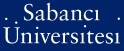# Solving stochastic mathematical programs with complementarity constraints using simulation

Birbil, Ş. İlker and Gürkan, Gül and Listeş, Ovidiu (2006) Solving stochastic mathematical programs with complementarity constraints using simulation. Mathematics of operations research, 31 (4). pp. 739-760. ISSN 0364-765XPDF - Registered users only - Requires a PDF viewer such as GSview, Xpdf or Adobe Acrobat Reader401Kb

Official URL: http://dx.doi.org/10.1287/moor.1060.0215

## Abstract

We consider stochastic mathematical programs with complementarity constraints, in which both the objective and constraints involve limit functions that need to be approximated. Such programs can be used for modeling “average” (expected) or steady-state behavior of complex stochastic systems. We first describe these stochastic mathematical programs with complementarity constraints and compare them with di erent stochastic mathematical programs with equilibrium constraints from the literature. This explicit discussion may facilitate selecting an appropriate stochastic model. We then describe a simulation-based method called sample-path optimization for solving these problems and provide su cient conditions under which appropriate approximating problems will have solutions converging to a solution of the original problem almost-surely. We illustrate an application on toll pricing in transportation networks. We explain how uncertainty can be incorporated and the approximating problems are solved using an o -the-shelf solver. These developments enable solving certain stochastic bilevel optimization problems and Stackelberg games using simulation.

Item Type: Article stochastic mathematical programs with complementarity constraints; simulation; stability; mathematical programs with equilibrium constraints; stochastic network equilibrium; toll pricing problem Q Science > QA Mathematics 223 Ş. İlker Birbil 08 Feb 2007 02:00 17 Sep 2019 13:56

Repository Staff Only: item control page